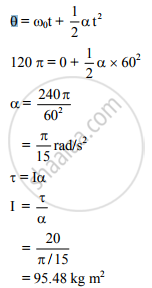HSC Science (Computer Science) 12th Board ExamMaharashtra State Board
Share

A Body Starts Rotating from Rest. Due to a Couple of 20 Nm It Completes 60 Revolutions in One Minute. Find the Moment of Inertia of the Body. - HSC Science (Computer Science) 12th Board Exam - Physics

ConceptPhysical Significance of M.I (Moment of Inertia)

Question

A body starts rotating from rest. Due to a couple of 20 Nm it completes 60 revolutions in one minute. Find the moment of inertia of the body.

Solution

T = 20 N m, n = 60 revolutions in 60 s = 1 r.p.s., t = 1 min = 60 s
Moment of inertia (I) = ?

Angular displacement θ = ωt = 2πnt
= 2π × 1 × 60
= 120 π rad∴ Moment of inertia of the body is 95.48 kg m2

Is there an error in this question or solution?

APPEARS IN

2013-2014 (October) (with solutions)
Question 4.2 | 7.00 marks
Solution A Body Starts Rotating from Rest. Due to a Couple of 20 Nm It Completes 60 Revolutions in One Minute. Find the Moment of Inertia of the Body. Concept: Physical Significance of M.I (Moment of Inertia).
S